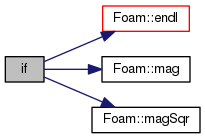The OpenFOAM Foundation
temperatureAndPressure.H File Reference

Accumulates values for temperature and pressure measurement, and calculates and outputs the average values at output times. Requires temperatureAndPressureVariables.H to be declared before the timeloop. More...

Go to the source code of this file.

## Functions

if (runTime.writeTime())

## Detailed Description

Accumulates values for temperature and pressure measurement, and calculates and outputs the average values at output times. Requires temperatureAndPressureVariables.H to be declared before the timeloop.

Original source file temperatureAndPressure.H

Definition in file temperatureAndPressure.H.

## Function Documentation

 if ( runTime. writeTime() )

Definition at line 48 of file temperatureAndPressure.H.

Here is the call graph for this function:## Variable Documentation

 accumulatedTotalLinearMomentum = singleStepTotalLinearMomentum

Definition at line 32 of file temperatureAndPressure.H.

Referenced by if().

 accumulatedTotalMass = singleStepTotalMass

Definition at line 34 of file temperatureAndPressure.H.

Referenced by if().

 accumulatedTotalLinearKE = singleStepTotalLinearKE

Definition at line 36 of file temperatureAndPressure.H.

Referenced by if().

 accumulatedTotalAngularKE = singleStepTotalAngularKE

Definition at line 38 of file temperatureAndPressure.H.

Referenced by if().

 accumulatedTotalPE = singleStepTotalPE

Definition at line 40 of file temperatureAndPressure.H.

Referenced by if().

 accumulatedTotalrDotfSum = singleStepTotalrDotf

Definition at line 42 of file temperatureAndPressure.H.

Referenced by if().

 accumulatedNMols = singleStepNMols

Definition at line 44 of file temperatureAndPressure.H.

Referenced by if().

 accumulatedDOFs = singleStepDOFs

Definition at line 46 of file temperatureAndPressure.H.

Referenced by if().